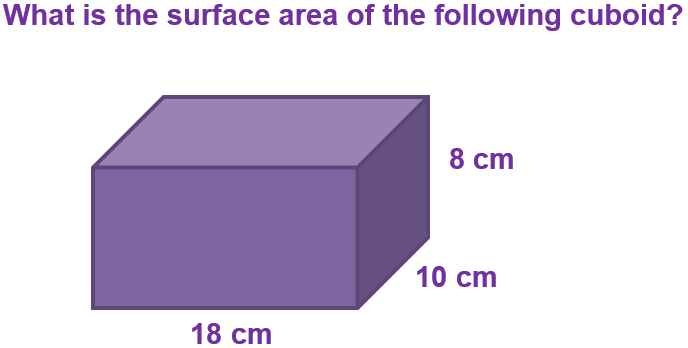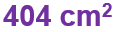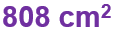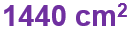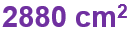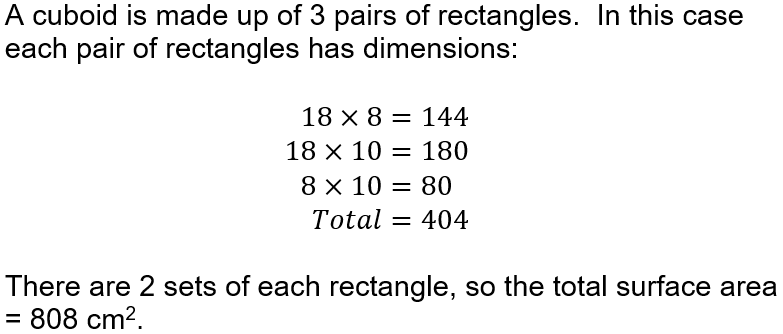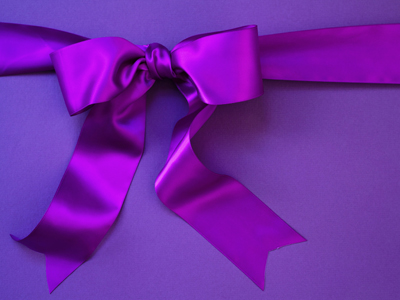What connects a birthday present to a maths quiz about surface area? Play the quiz and find out...

# Surface Area (F)

We know that two-dimensional shapes have area, which is the amount of space the shape takes up. The equivalent for three-dimensional shapes is volume. But every three-dimensional shape is made up of surfaces, and these surfaces will each have their own area. To imagine this, think of folding open a box so that it can be laid flat (this is called the NET of the box). This GCSE Maths quiz will see how well you can visualise and work with surface areas of three-dimensional shapes.

Trying to visualise three-dimensional shapes can be a tricky concept. One way to try to understand them is to picture unfolding it to form the net of the shape. Cubes and cuboids will form a series of rectangles, while a cylinder will have one rectangle, with a circle at each end. Pyramids will have a base that could be a triangle, square or any other polygon, plus a number of triangles that all fold up to form the top of the pyramid. A cone is a circle for the base, plus a sector for the curved surface.

Once you can picture the net of a three-dimensional shape, you can start to work out its surface area. It will involve at least 2 calculations, often many more, and so it is important to explain what each calculation is working out. You don’t have to use sentences; words or even well-drawn symbols can help communicate your strategy.

Question 1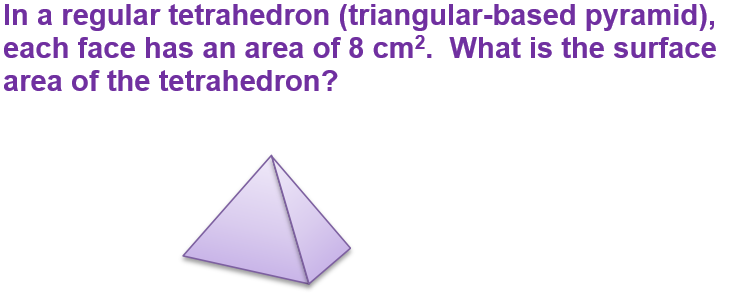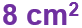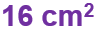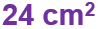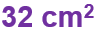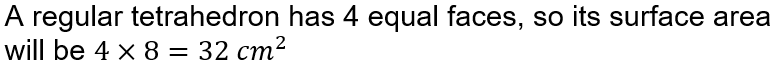Question 2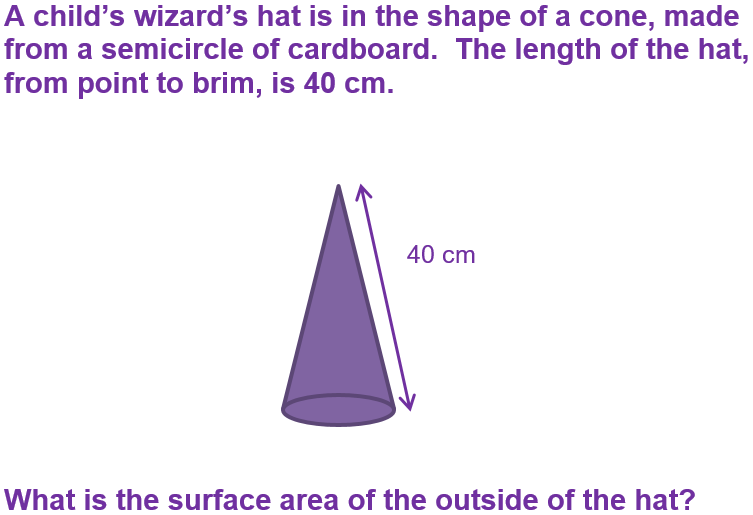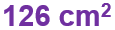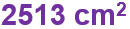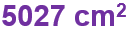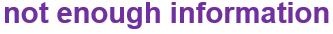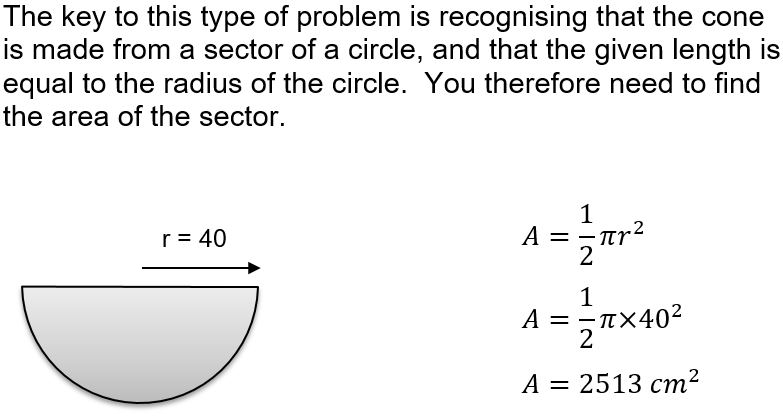Question 3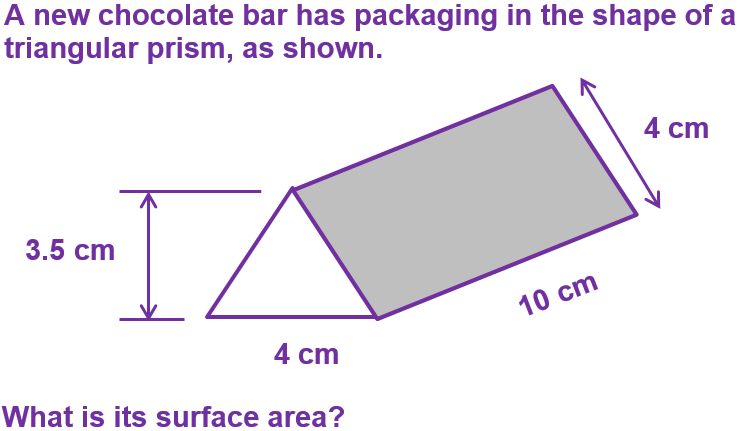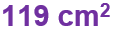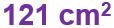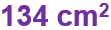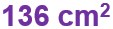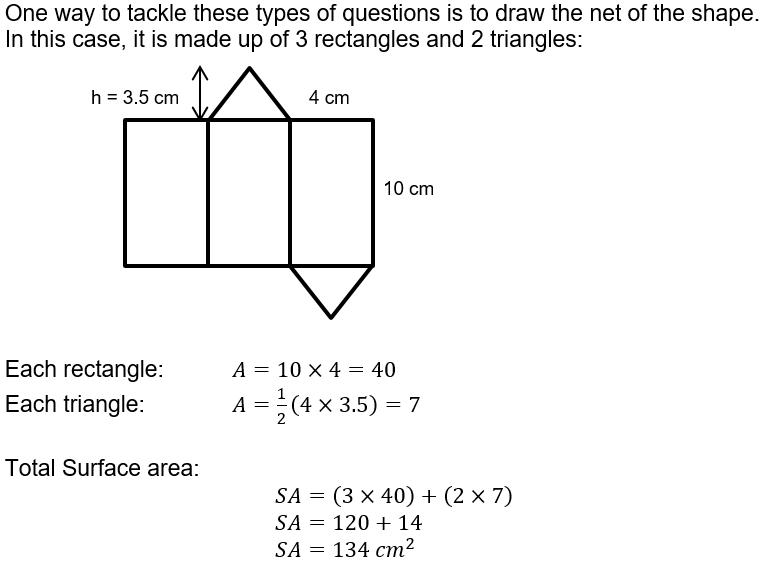Question 4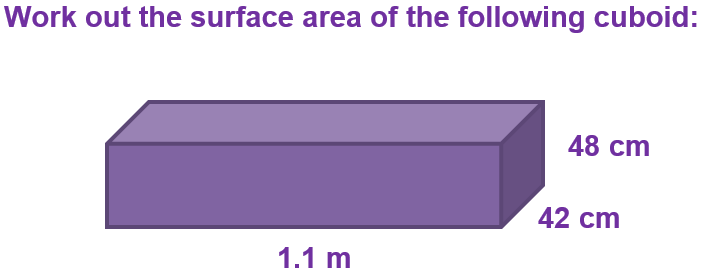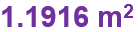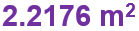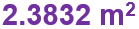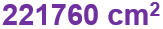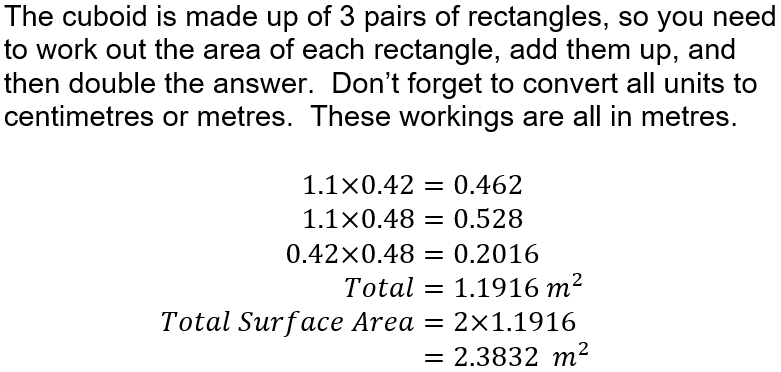Question 5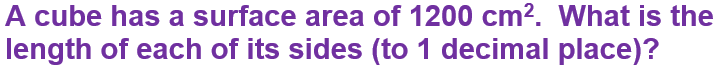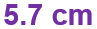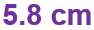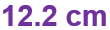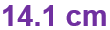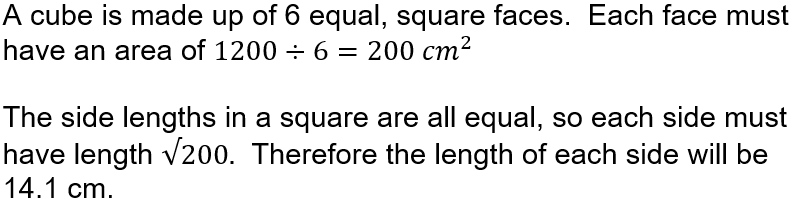Question 6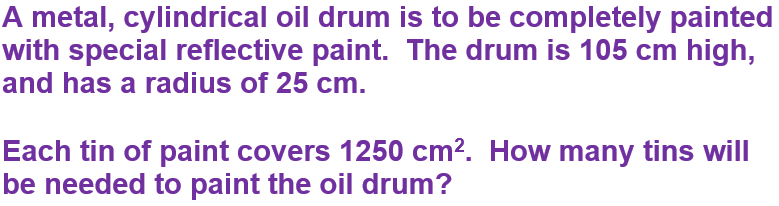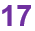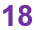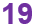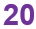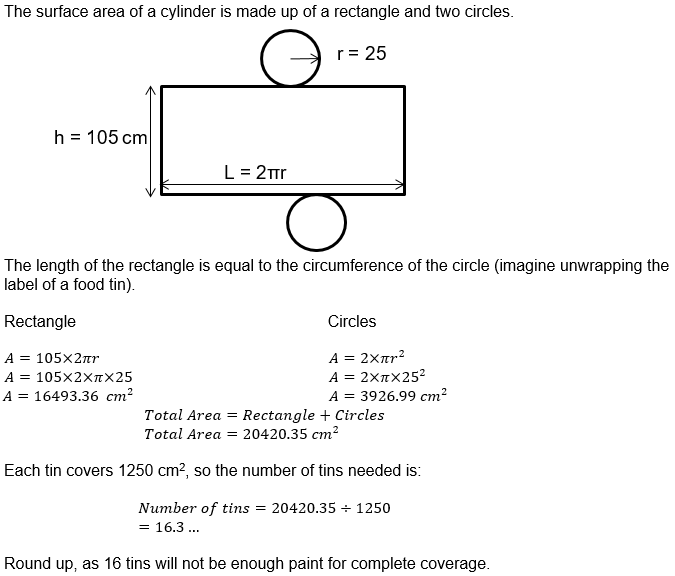Question 7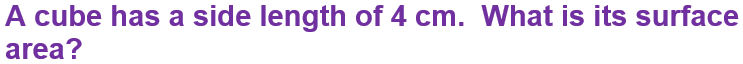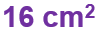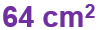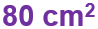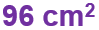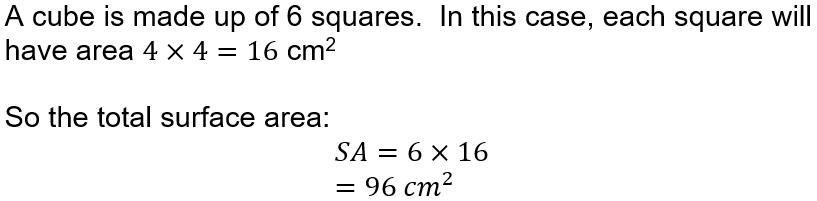Question 8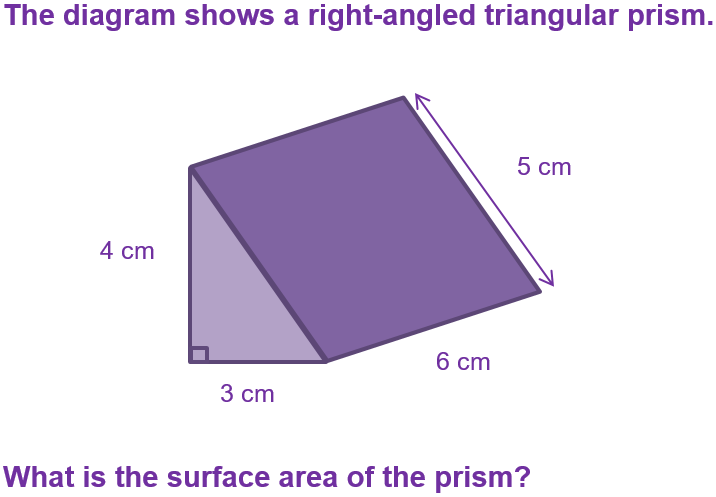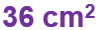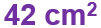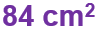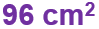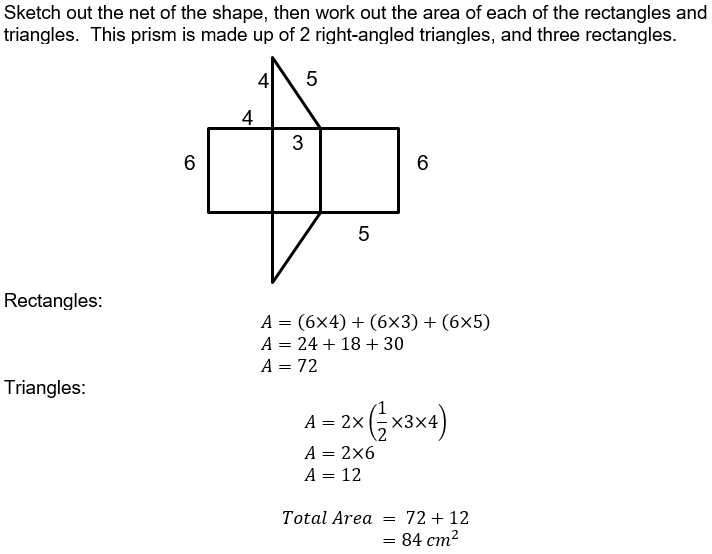Question 9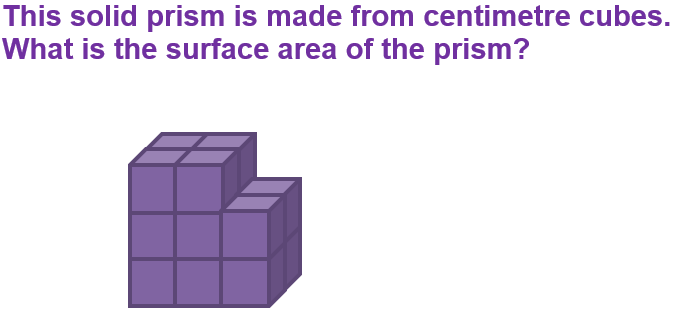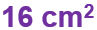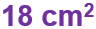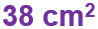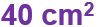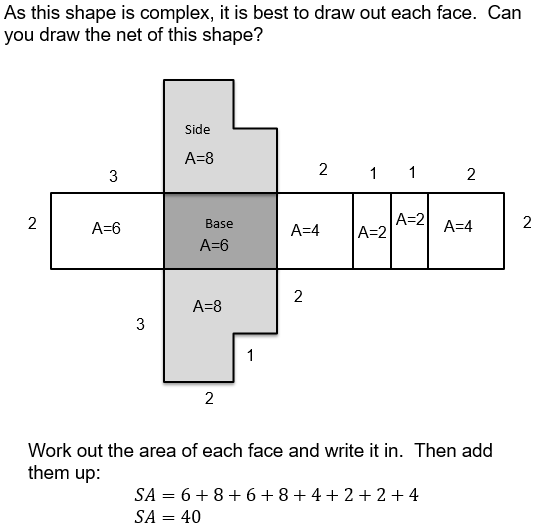Question 10